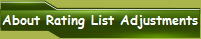Comparing the ratings of chess computers to humans has always been tricky because of the single formula used in rating calculators do not allow the calculator to follow the rules of the two formulas required to convert a USCF rating to a FIDE rating or a FIDE rating to a USCF rating. This makes a comparison to USCF or FIDE player ratings very difficult. The United States Chess Federation (USCF) states in all cases an entry level rating for a beginner as: “Use an initial rating of 750 based on 0 games”. Even the weakest chess computers know the basic values of each chess piece and as a result avoid giving up a higher valued piece for a lesser valued piece. Therefore it is unlikely for even the very weakest chess computer to be rated below an initial beginner rating of ELO 750 as suggested by USCF with this Beginner start value. OLD USCF FORMULA TO CONVERT A FIDE RATING TO USCF RATING FOR LESS THAN 2000 USCF = 720 + 0.625*FIDE if FIDE < 2000 The above old rating conversion from FIDE to USCF works as follows, if for example you have a FIDE rating of 1950 ELO: 720+(0.625*1950) = USCF 1939 ELO You can also by reversing the calculation, use the following formula convert a USCF rating to FIDE: FIDE = USCF - 720 / 0.625 Therefore if for example you start with a USCF rating 1939 ELO and you want to convert it to FIDE you use: (1939-720)/0.625 = FIDE 1950 ELO Now by using the same above old USCF formula lets try and convert SciSys Mini Chess that is rated by Schachcomputer.Info Active Rating List as ELO 709. Let’s do this with the two assumptions that ELO 709 equals     1) 709 FIDE and 2) 709 USCF: 720+(0.625*709) = USCF 1163 ELO (709-720)/0.625 = FIDE - 18 ELO Obviously when you compare the results of this old USCF Formula you see it was not very good at calculating a rating for players in the absolute beginner ranges as in the above examples where a 709 rated USCF player has a FIDE rating of (minus) -18 ELO. Even reversing as in example 2 where the FIDE Rating of ELO 709 equals a USCF rating of 1163 ELO is not very realistic. This old formula was probably never meant to go below converting a 1400 ELO FIDE player to USCF.  Which becomes obvious when you realize that FIDE ratings only start at 1400 ELO for Novices. Nothing below 1400 ELO exists for FIDE. OLD USCF FORMULA TO CONVERT A FIDE RATING TO USCF RATING FOR MORE THAN 2000 USCF = -350 + 1.16*FIDE if FIDE >= 2000 The formula for over 2000 ELO players is a little more realistic. For example a FIDE 2050 ELO player would have: -350+(1.16*2050) = USCF 2028 ELO However, even this rating was not too accurate as it is generally accepted based on comparing true ratings of players who have both FIDE and USCF that at around the 2000 ELO level a USCF player should be approximately 60 ELO higher.  Therefore the 2050 FIDE players should have approximately the equivalent USCF rating of 2110 ELO. Therefore you can quickly see a large discrepancy between 2028 ELO and 2110 ELO of 88 ELO.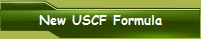A new USCF Rating System formula was reported by USCF on June 1, 2015: If an unrated player has a FIDE rating, use a converted rating according to the following formula: US rating = 180 + 0.94×FIDE if FIDE ≤ 2000 US rating = 20 + 1.02×FIDE if FIDE > 2000   NEW USCF FORMULA TO CONVERT A FIDE RATING TO USCF RATING FOR MORE THAN 2000 US rating = 20 + 1.02×FIDE if FIDE > 2000 For over 2000 rated players the formula 20 + 1.02×FIDE if FIDE > 2000 works really well as the chart below shows: FIDE 2000 ELO = USCF ELO 2060 (+60 Points) FIDE 2100 ELO = USCF ELO 2162 (+62 Points) FIDE 2200 ELO = USCF ELO 2264 (+64 Points) FIDE 2300 ELO = USCF ELO 2366 (+66 Points) FIDE 2400 ELO = USCF ELO 2468 (+68 Points) FIDE 2500 ELO = USCF ELO 2570 (+70 Points) FIDE 2600 ELO = USCF ELO 2672 (+72 Points) FIDE 2700 ELO = USCF ELO 2774 (+74 Points) FIDE 2800 ELO = USCF ELO 2876 (+76 Points) FIDE 2900 ELO = USCF ELO 2978 (+78 Points) FIDE 3000 ELO = USCF ELO 3080 (+80 Points) There is a graduated addition of 1 point added to the initial 60 points difference for every 50 point FIDE rate increase. You can also use this formula in reverse to find the FIDE equivalent rating of a USCF rated player: FIDE rating =(USCF-20)/1.02 Using the above formula, a USCF 2050 ELO rated player would have a FIDE rating of 1990 ELO. For players rated over 2000 ELO this new USCF formula seems to work very well.   NEW USCF FORMULA TO CONVERT A FIDE RATING TO USCF RATING FOR LESS THAN 2000 US rating = 180 + 0.94×FIDE if FIDE ≤ 2000 Taking the above formula for a FIDE 1999 ELO player you would obtain a USCF rating of ELO 2059. This seems to work well for a FIDE 1999 ELO player. However lets take the same beginner computer, SciSys Mini Chess that is rated in Schachcomputer.Info Active Rating List as ELO 709. Let’s do this with the same two assumptions that ELO 709 equals     1) 709 FIDE and 2) 709 USCF: 180+(0.94*709) = USCF 846 ELO (709-180)/0.94 = FIDE 563 ELO Logically, since FIDE ratings start at Novice 1400 ELO and an initial USCF rating for a Beginner starts at 750 ELO, neither of the above values are accurate as there has to be a natural progression between FIDE and USCF ELO conversion. The value of USCF 846 is too high for USCF as it would be impossible for USCF to be rated higher than FIDE at this absolute beginner rating scale. The same applies to the value of FIDE 563 which is too low as it would be impossible for FIDE to be rated lower than USCF at this absolute beginner rating scale. A recent Chess.Com report provides some important insights and validation that there does indeed seems to be a natural progressive conversion between FIDE and USCF players.  The chart below shows this. Chess.Com had carried out a survey where its members who had both USCF and FIDE ratings were compared together with their performance ratings at Online Blitz, Bullet and Turn Based chess.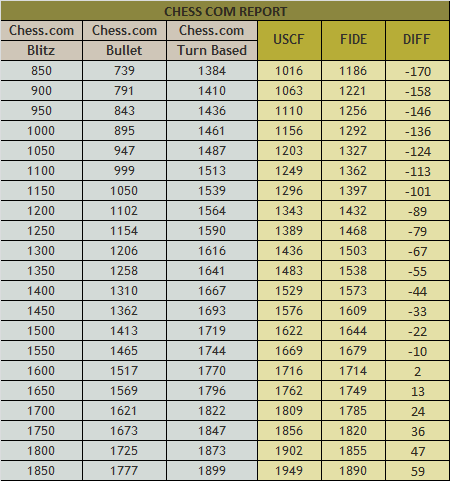If you study the above chart, you will see that there is a consistent 1 point FIDE loss for every 4 points of USCF from a starting point of FIDE 1886 ELO, where FIDE is rated lower than USCF by 60 Points. With this new understanding I was able to create a new formula to accurately convert a FIDE rated player below 1900 FIDE ELO to a USCF rating. The formula is:  US rating = -570 + FIDE/0.75 if FIDE ≤ (EQUAL OR LESS THAN) 1886 or FIDE rating = 0.75 * 570 + USCF if USCF ≤ (EQUAL OR LESS THAN) 1945 Here are some example FIDE player conversions using the above formula: FIDE 1885 ELO PLAYER = USCF 1943 ELO = +58 ELO FIDE 1800 ELO PLAYER = USCF 1830 ELO = +30 ELO FIDE 1600 ELO PLAYER = USCF 1563 ELO = -37 ELO FIDE 1400 ELO PLAYER = USCF 1296 ELO = -104 ELO FIDE 1200 ELO PLAYER = USCF 1030 ELO = -170 ELO FIDE 1000 ELO PLAYER = USCF 763 ELO = -237 ELO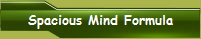The official new USCF formula (US rating = 20 + 1.02×FIDE if FIDE > 2000) for FIDE over 2000 ELO rated players works well. But you do have to lower it for use with FIDE player ratings of FIDE > (over) 1886 ELO Therefore taking this into consideration in order to more accurately compare a Chess Computer rating list with a human rating list you have to use the following formulas if you begin with a FIDE start value to convert to a USCF rating: US rating = 20 + 1.02×FIDE if FIDE > 1886 US rating = -570 + FIDE/0.75 if FIDE ≤ 1886 The official USCF conversion formula is used with only a minor adjustment where it is implemented down to 1945 ELO instead of 2000 ELO. The following new progressive adjustment formula is used for below 1945 ELO. If you have a USCF start value you can reverse the formulas as follows to get a FIDE rating: FIDE rating = USCF-20 / 1.02 if USCF > 1945 FIDE rating = 0.75 * 570 + USCF if USCF ≤ 1945 Now in order for human’s to get a better idea of how dedicated computers compare to their own playing levels across all rated dedicated computers from strongest to absolute weakest, I have taken Schachcomputer.Info’s Active Rating list with the assumption that the first computer on that list Tasc R40 V2.5 has a fairly accurate FIDE rating of ELO 2405 (European Player rating). Taking the above over Official USCF formula, Tasc R40 V2.5 USCF rating would be USCF 2473 ELO. This follows exactly the official USCF rating conversion formula resulting in a difference of + 68 ELO. This 68 ELO adjustment was made across all Schachcomputer.Info rated computers resulting in the lowest rated computer having a USCF ELO of 777. This in turn also matches the USCF minimum start rating for a human of 750 ELO. 777 ELO, being slightly higher than a human also makes sense. To create the FIDE ratings these previously explained formulas were used: FIDE rating = USCF-20 / 1.02 if USCF > 1945 FIDE rating = 0.75 * 570 + USCF if USCF ≤ 1945 Below you can see the result of this exercise which accurately shows Schachcomputer.Info’s rating and compares this with how it would look like for a human player FIDE or USCF player to reference his playing strength against dedicated chess computers, which not only exactly matches USCF ratings conversions at FIDE 1886 ELO and above but also acknowledges the logic of USCF and FIDE start ratings and Chess.com findings based on human rated players.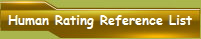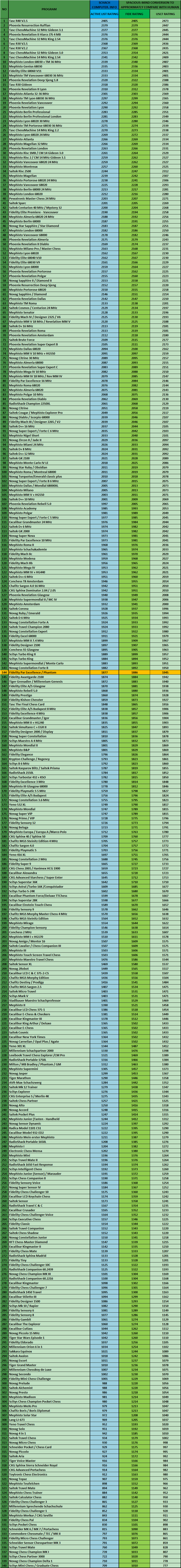To view the Schachcomputer.Info Active Chess Computer Rating List click here Well that was a fun exercise!
 [Home] [Collection] [Tournaments] [Tests] [Computers] [What's New] [Links]